1. Define tensile stress and tensile strain.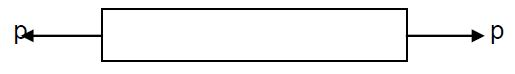The stress induced in a body, when subjected to two equal and opposite pulls, as a result of which there is an increase in length, is known as tensile stress.

The ratio of increase in length to th original length is known as tensile strain.

2. Define compressive stress and compressive strain.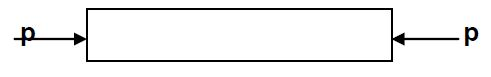The stress induced in a body, when subjected to two equal and opposite pushes, as a result of which there is an decrease in length, is known as compressive stress.

The ratio of increase in length to th original length is known as compressive strain.

3. Define shear stress and shear strain.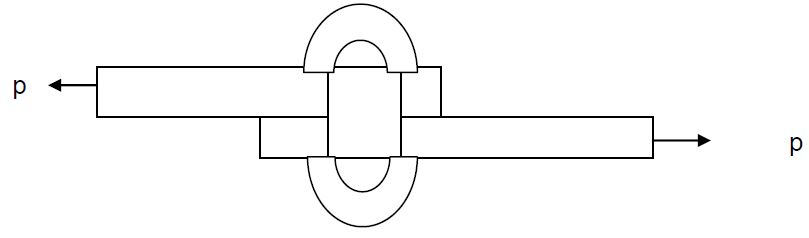The stress induced in a body, when subjected to two equal and opposite forces, which are acting tangentially across the resisting section as a result of which the body tends to shear off across the section is known as shear stress and corresponding strain is known as shear strain.

4. Sketch a composite bar.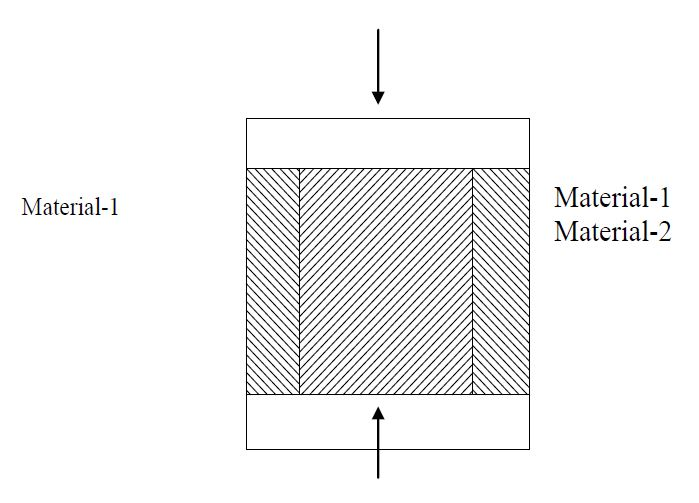A bar made up of two or more bars of equal length but of different materials rigidly fixed with each other behaving as one unit for extension or for compression when subjected to an axial tensile or compressive load is called as a composite bar.

5. Give example for ductile, brittle and malleable materials.

Ductile materials     ------------> steel, copper

Brittle materials       ------------> wrought iron

Malleable materials ------------> cast iron

6. Define Poisson’s ratio

The ratio of lateral strain to the linear strain is a constant for a given material, when the material is stressed within the elastic limit. This ratio is Poisson’s ratio and it is generally denoted by 1/m or µ.

Poisson’s ratio 1/m = µ = linear starin/ lateral starin.

7. Write the relationship between modulus of elasticity, modulus of rigidity and Poisson’s ratio.

The relationship between modulus of elasticity, modulus of rigidity and Poisson’s ratio is given by

E = 2C (1+ 1/m)

E=Modulus of elasticity

C=Modulus of rigidity

1/m = Poisson’s ratio

8. If the strain in three mutually perpendicular directions are ex, ey and ez then what is the value of volumetric strain?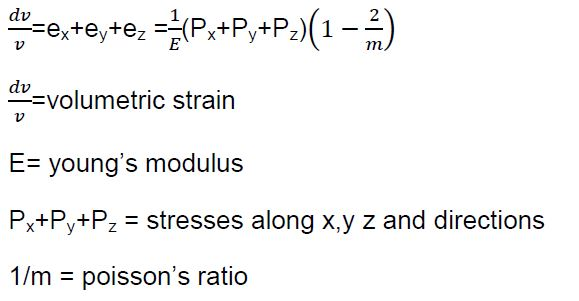9. State Hooke’s law.

Hooke’s law is stated as when a material is loaded within elastic limit, the stress is proportional to the strain produced by stress, or Stress/strain=constant. This constant is termed as modulus of elasticity.

10. Define stress and strain.

Stress: The force of resistance per unit area, offered by a body against deformation is known as stress.

Mathematically stress is written as

f=P/A

Where f= stress

A=cross-sectional area.

Unit of stress: stress is represented in N/m2

Strain:

The ratio of change in dimension to the orginal dimension when subjected to an external load is termed as strain and is denoted by e. It has no unit.

11. Define modulus of rigidity

The ratio of shear stress to the corresponding shear strain when the stress is within the elastic limit is known as modulus of rigidity or shear modulus and is denoted by C or Gor N

Shear stress / Shear strain = q / ?

12. Define modulus of elasticity.

The ratio of tensile stress or compressive stress to the corresponding strain is known as modulus of elasticity or young’s modulus and is denoted by E.

stress / strain  = E

13. Define Bulk modulus.

When a body is subjected to an uniform direct stress in all the three mutually perpendicular directions, the ratio of the direct stress to the corresponding volumetric strain is found to be a constant is called as the bulk modulus of the material and is denoted by K.

K =  direct stress / volumetric strain = P/A / dv/v

14. Define longitudinal strain and lateral strain.

Longitudinal strain: longitudinal strain is defined as the deformation of the body per unit length in the direction of the applied load.

Longitudinal strain= dL/L

Where L= length of the body.

P= tensile force acting on the body

dL= increase in the length of the body in the direction of P

15. Draw the stress-strain curve for a

1. Ductile material

2. Brittle material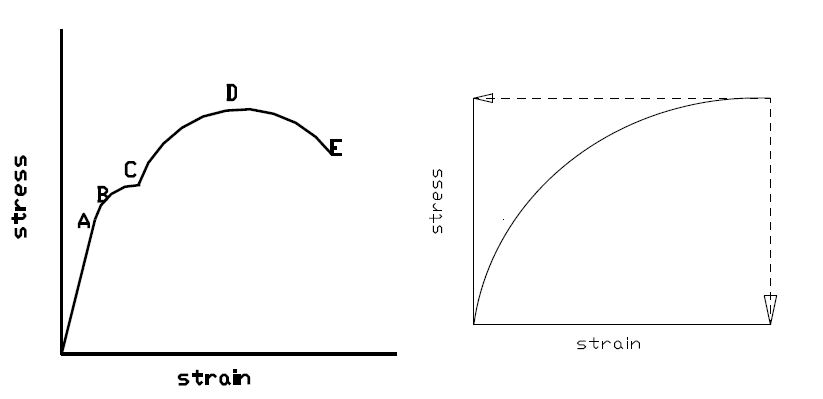16. Define factor of safety

It is defined as the of ultimate stress to the working stress or permissible stress.

Factor of safety = ultimate stress / permissible stress

17. State the two conditions employed in solving a composite bar subjected to an  axial load.

Two conditions employed in solving a composite bar are:

1. P=f1A1 + f2A2

2. e= f1/E1 + f2/E2

e= strain

f1 and f2 = stress set up in the respective materials.

E1 and E2 = modulus of elasticity of the respective materials.

Give the relationship between modulus of elasticity, modulus of rigidity and bulk modulus.

E = 9KC / 3K+C

Where

E= young’s modulus

K= bulk modulus

C= rigidity modulus

18. Give the relationship between modulus of elasticity, bulk modulus and poisson’s ratio.

E = 3K( 1 - 2/m )

Where

E = Young’s modulus

K = Bulk modulus

1 / m = Rigidity modulus

19. What is stability?

The stability may be defined as an ability of a material to withstand high load without deformation.